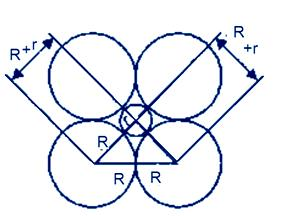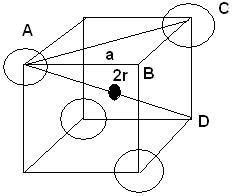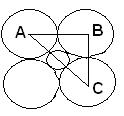Courses

# Test: Packing Efficiency

## 10 Questions MCQ Test Chemistry for JEE | Test: Packing Efficiency

Description
This mock test of Test: Packing Efficiency for JEE helps you for every JEE entrance exam. This contains 10 Multiple Choice Questions for JEE Test: Packing Efficiency (mcq) to study with solutions a complete question bank. The solved questions answers in this Test: Packing Efficiency quiz give you a good mix of easy questions and tough questions. JEE students definitely take this Test: Packing Efficiency exercise for a better result in the exam. You can find other Test: Packing Efficiency extra questions, long questions & short questions for JEE on EduRev as well by searching above.
QUESTION: 1

### For which type of cubic lattice, a = 2r?

Solution:

In simple cubic lattice the side of the solid structure is equal to twice the radius of atom. Hence the correct answer is simple cubic.

QUESTION: 2

### Packing efficiency of body centred cubic unit cell is:

Solution:

The packing efficiency of both types of close packed structure is 74%, i.e. 74% of the space in hcp and ccp is filled. The hcp and ccp structure are equally efficient; in terms of packing. The packing efficiency of simple cubic lattice is 52.4%. And the packing efficiency of body centered cubic lattice (bcc) is 68%.

QUESTION: 3

### Packing efficiency in a unit cell is never 100% because constituent particles are assumed to be:

Solution:

The constituent particles i.e. atoms, molecules and ions are assumed to be spheres.

QUESTION: 4

If r is radius of void and R is radius of the sphere, then for an atom to ocuppy an octahedral void which relation holds true?

Solution:Let take r and R are the radii of the octahedral site and atoms respectively,

then use Pythagoras theorem we get

(2R)2 = (R+r)2 +(R+r)2

4R2    = 2(R+r)2

Divide by 2 we get

2R2    = (R+r)2

Take root both side we get

R√2 =R+r

R√2 – R = r

r  = R(√2–1)

value of √2= 1.414

r  = R(1.414–1)

r  = 0.414 R

QUESTION: 5

Which type of void is represented by the following diagram?Solution:

A tetrahedral void is formed when one sphere or particle is placed in the depression formed by three particles.
Definition of Tetrahedral Void: The vacant space or void among the four constituent particles having tetrahedral arrangement in the crystal lattice is called tetrahedral void.

QUESTION: 6

HCP structure is present in:

Solution:
• Magnesium has hexagonal close-packed (HCP) crystal structure.
• Bonding is non-directional metallic bonding.
• Magnesium is metallic solid. The units occupying lattice sites are Mg ions and these ions are surrounded by mobile or delocalised electrons.
• The arrangement of ions in one plane the arrangement of ions is a hexagonal array or closed packed layer. Thus each metal ion is touching six adjacent ions in one plane.
QUESTION: 7

If r is the radius of the void and R is the radius of the sphere, what is AC in the following diagram?Solution:

AC= Diameter of void + (2× Radius of sphere) 》AC = 2r+2R =2 (R+r)

QUESTION: 8

Which of the following shows maximum packing efficiency?

Solution:

HCP= 74% packing efficiency
BCC= 68% packing efficiency
Simple cubic= 52.4% packing efficiency

QUESTION: 9

The number of atoms in each unit cell in ccp structure is:

Solution:

In ccp structure: number of atoms per unit cell is

(8 x 1/8)+(6 x 1/2)=1+3=4

So, number of atoms = 4.

QUESTION: 10

The number of atoms per unit cell in a body centred cubic arrangement is:

Solution:

For a bcc unit cell, number of atoms per unit cell = (8 *1/8) + 1 = 2